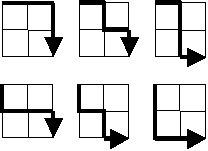# Project Euler #015

Starting in the top left corner of a 2 × 2 grid, there are 6 routes (without backtracking) to the bottom right corner.How many routes are there through a 20 × 20 grid?

``````let rec find_path square_size x y =
let complete = x = square_size || y = square_size
match complete with
| true -> 1L
| false -> (find_path square_size (x + 1) y) + find_path square_size x (y + 1)

// This will take some time
find_path 20 0 0
``````